GMAT Math : Solving equations

Example Questions

1 2 3 4 5 6 8 Next →

Example Question #71 : Equations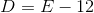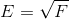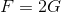Express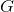in terms of.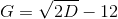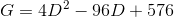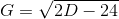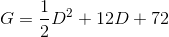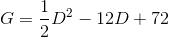Explanation: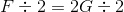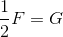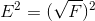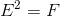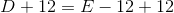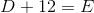By two substitutions: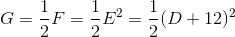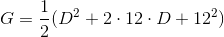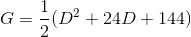Example Question #72 : Equations

Which of the following is the solution set of the equation: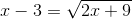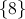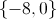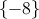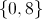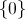Explanation:

Square both sides of the equation, then solve the resulting quadratic equation by factoring: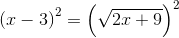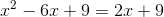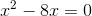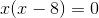Eitheror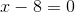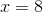Checking both solutions, however: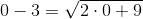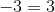This eliminates 0 as a solution.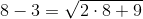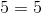8 turns out to be the only solution.

1 2 3 4 5 6 8 Next →

Tired of practice problems?

Try live online GMAT prep today.